Back to overview

# 3. Visualization of data

This document lists and illustrates a number of basic plot functions. For replicating the example scripts, please load the restructured data set `AirPassengers` (see `help(AirPassengers)`) in R:

``load('dat-AirPassengers.rda')``

## BASIC PLOT FUNCTIONS

#### plot

The function `plot` sets up a plotting grid. The function optionally adds data in the form of a line or points, depending on the argument `type`. If you just would like to set up an empty grid and add data later, you could use the argument `type='n'`. Here are some examples:

``````dat\$Time <- dat\$Year+dat\$Month/12

# by default, type is set to 'p': points
plot(x=dat\$Time, y=dat\$AirPassengers)``````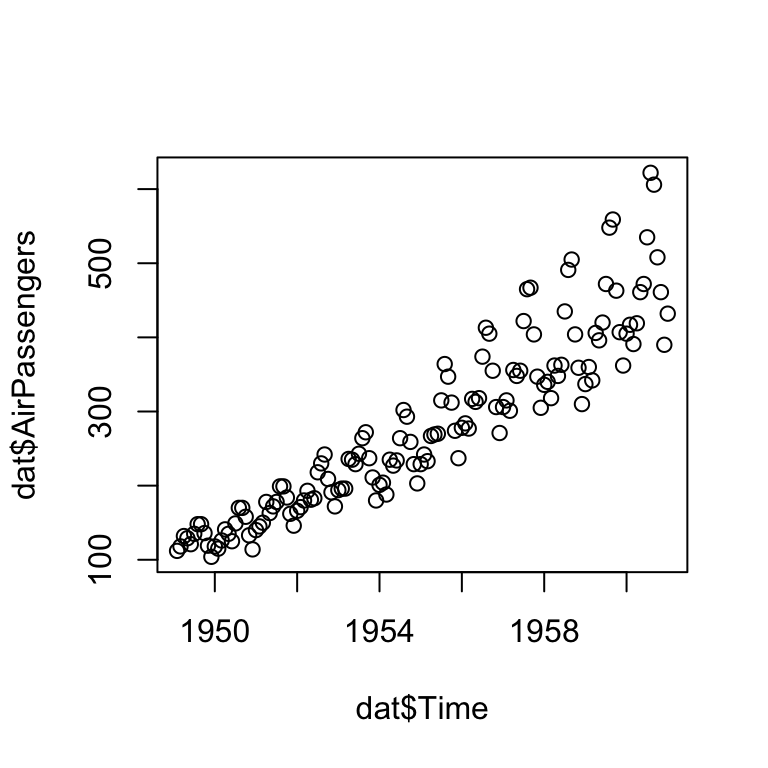``````# ... but lines may be more insightful here:
plot(x=dat\$Time, y=dat\$AirPassengers, type='l',
main="Title", xlab="Year", ylab="AirPassengers")``````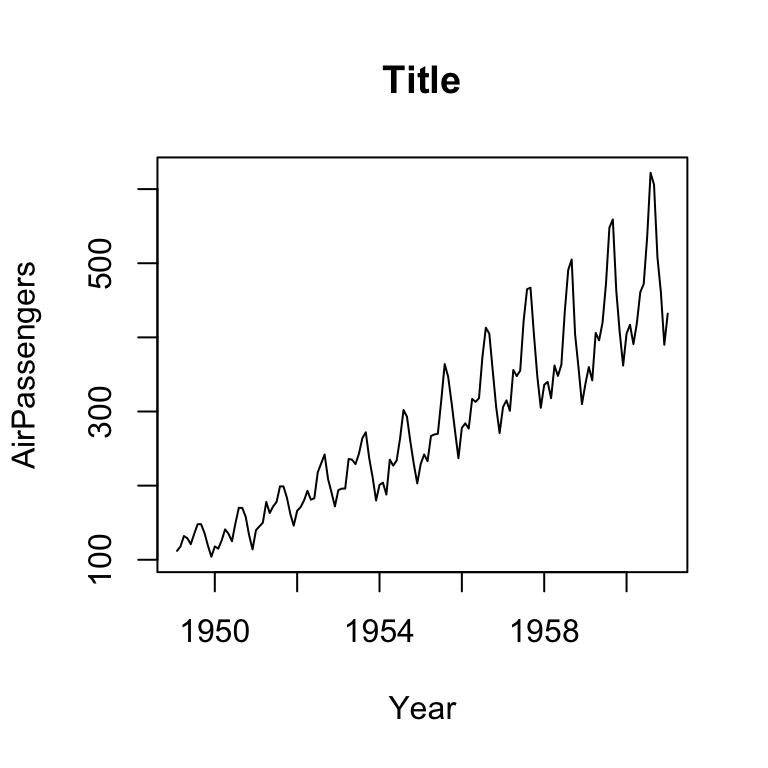``````# we could also set up an empty plot, to add points or lines later:
plot(x=c(), y=c(), type='n',
xlim=range(dat\$Time), ylim=range(dat\$AirPassengers),
main="Title", xlab="Year", ylab="AirPassengers",
bty='n') # <- no box around plot``````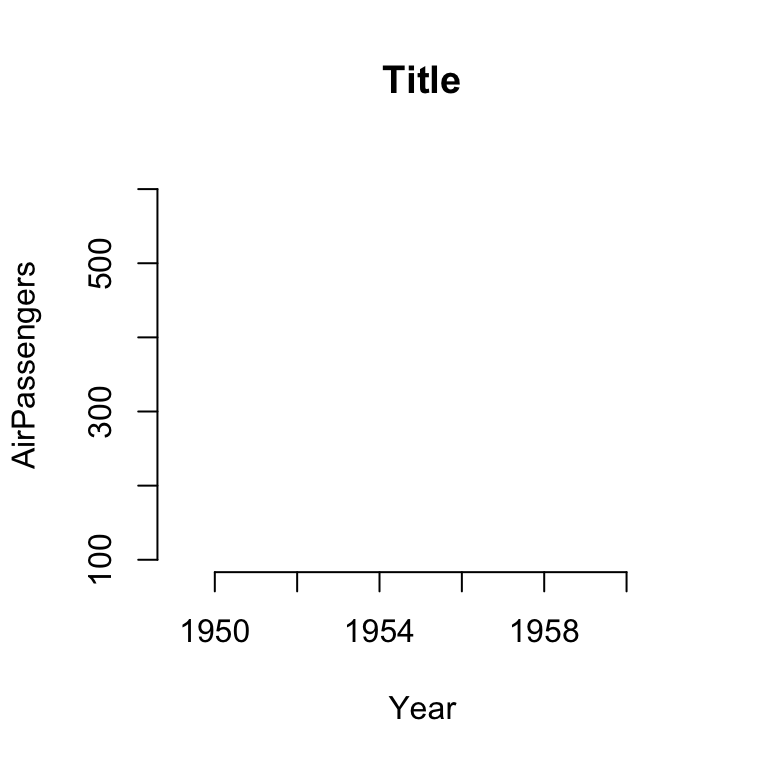``````# the library plotfunctions provides a wrapper for doing this:
library(plotfunctions)
# note that we set the *range* rather than actual values here
emptyPlot( range(dat\$Time), range(dat\$AirPassengers)) ``````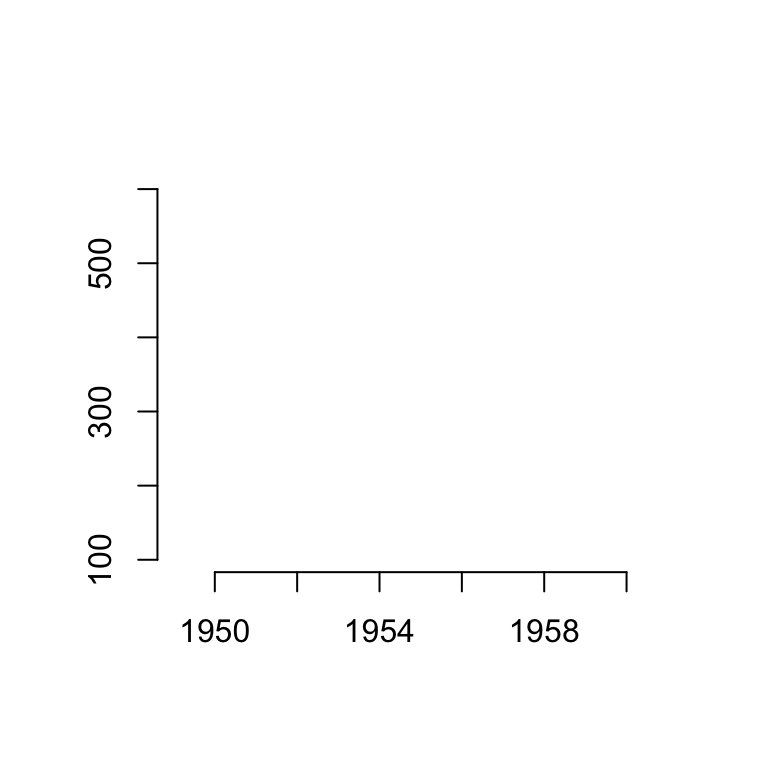``# main, xlab, and ylab can be also included as arguments (and many others) ``

See `help(par)` for graphical parameters that can be added to most plotting functions to change the layout and colors.

#### points

The function `points` is used to add points to a plot grid. The argument `pch` specifies the layout.

``````emptyPlot( range(dat\$Time), range(dat\$AirPassengers))
points(dat\$Time, dat\$AirPassengers,
pch=16, col=alpha(2)) # <- alpha() from package plotfunctions ``````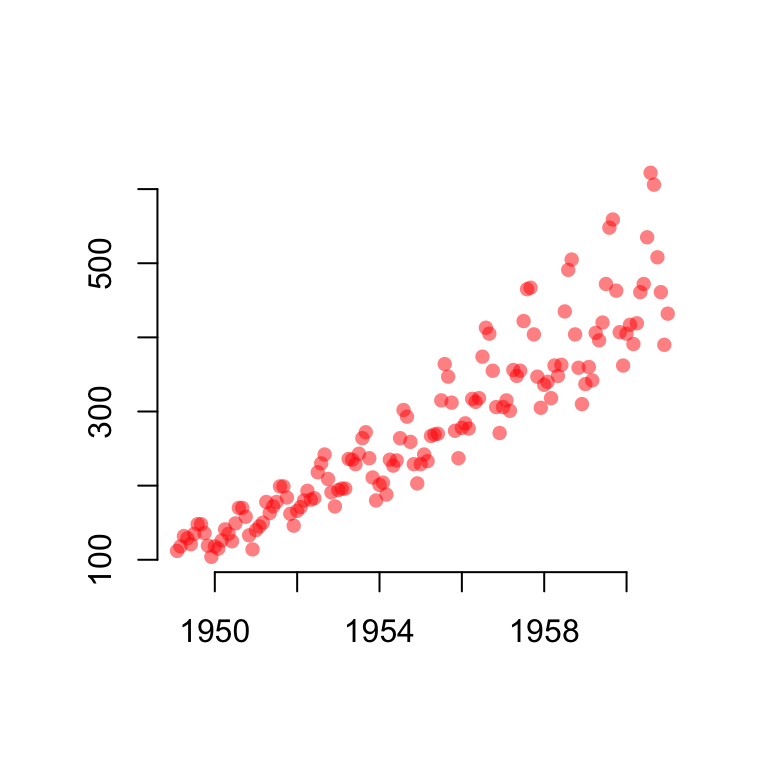``                             # could be used to adjust transparency of colors``

Various types of points:

``````par(cex=1.1)
emptyPlot(c(0,6), c(0,6), axes=FALSE, bty='o')
for(i in 1:5){
for(j in 1:5){
points(i, j, pch=(i-1)*5+j, bg=alpha(2))
text(i,j,labels=(i-1)*5+j, cex=.5, pos=3, col='red')
}
}``````